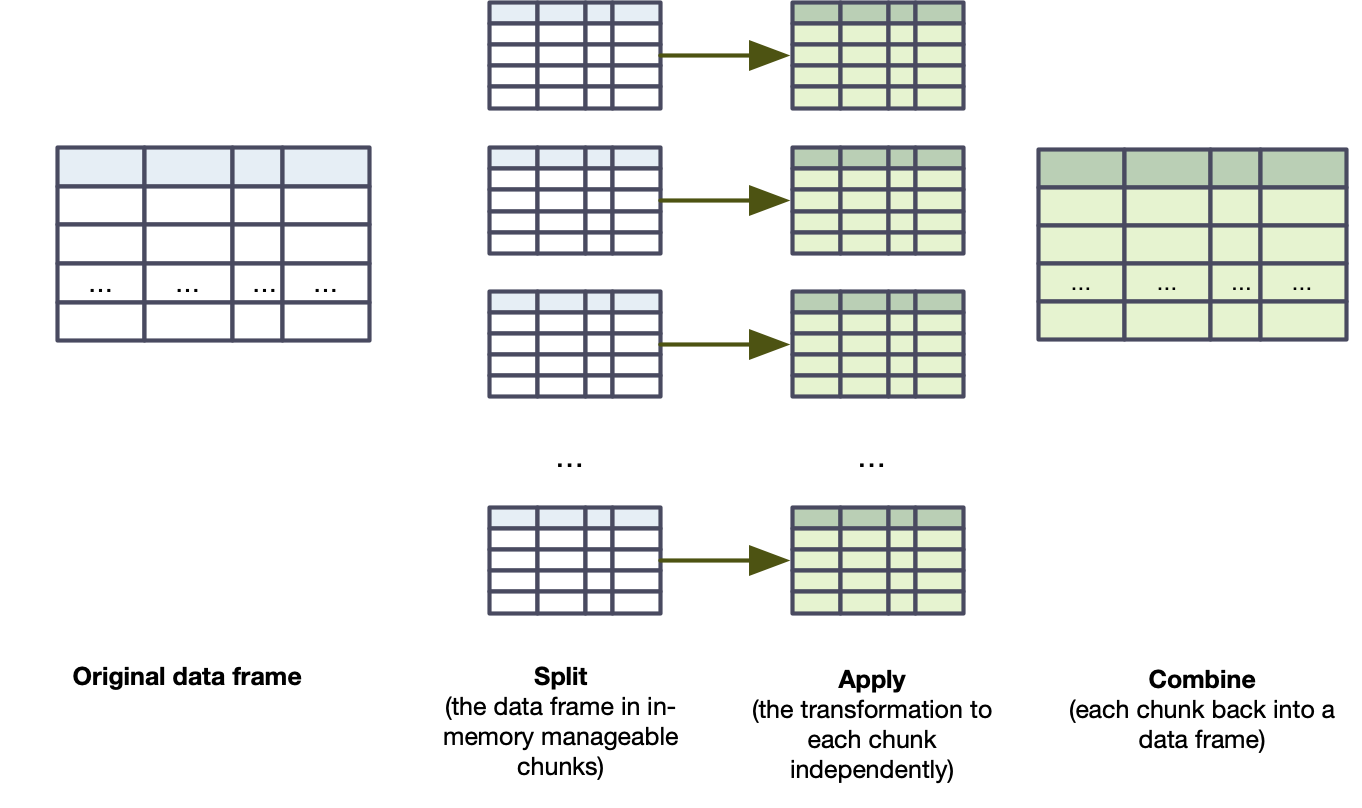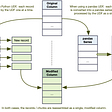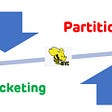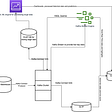# Big Data is Just a Lot of Small Data: using pandas UDF, part 2

· Using pandas Series UDF to accelerate column transformation compared to Python UDF.

· Addressing the cold start of some UDF using Iterator of Series UDF.

Take 37% off Data Analysis with Python and PySpark by entering fccrioux into the discount code box at checkout at manning.com.

## UDF on grouped data: aggregate and apply

This section covers UDF in the case where you need to worry about the composition of the batches. This is useful in two cases. For completion, I provide the common names used in Spark 3.:

1. Group aggregate UDF: you need to perform aggregate functions such as `count()` or `sum()`, as we saw in chapter 5.
2. Group map UDF: your data frame can be split into batches based on the values of certain columns, you then apply a function on each batch as if it was a Pandas data frame before combining each batch back into Spark data frame. For instance, we could want out `gsod` data to be batched by station-month and perform operations on the resulting data frames.

Both group aggregate and group map UDF are PySpark’s answer to the split-apply-combine pattern. At the core, split-apply-combine is just a series of three steps that are frequently used in data analysis.

1. First, you split your data set into logical batches (using `groupby()`).
2. You then apply a function to each batch independently.
3. Finally, you combine the batches into a unified data set.

To be perfectly honest, I did not know this pattern’s name until somebody pointed at my code one day and said “this is some nice split-apply-combine work you did there”. You probably use it intuitively as well. In PySpark’s world, I see it more as a divide and process move, as illustrated in figure 4.Figure 1. Split-apply-combine depicted visually. We batch/group the data frame, process each one with pandas, before unifying them into a (Spark) data frame again.

This section will cover each use-case, illustrate how they relate to the split-apply-combine pattern and introduce the relevant type of UDF. While they are clubbed in the same family and both work on `GroupedData` objects (seem in chapter 5 when we review the `groupby()` method), their syntax and usage are quite different.

With great power comes great responsibility: when grouping your data frame, make sure each batch/group is “Pandas-size”, i.e. it can be loaded comfortably in memory. If one or more batches is too big, you’ll get an out-of-memory exception.

## Group aggregate UDF

Only available from Spark 2.4+. Spark 2.4 need provide a `functionType` or `PandasUDFType.GROUPED_AGG` (from `pyspark.sql.functions`, see https://spark.apache.org/docs/2.4.0/sql-pyspark-pandas-with-arrow.html)

This section covers the group aggregate UDF, also known as the `Series to Scalar UDF`. With such a name, we already can imagine that it shares some affinities with the Series to Series UDF. Unlike the Series to Series, the group aggregate UDF distills the Series received as input to a single value. In the split-apply-combine pattern, the apply stage collapses the batches into a single record based on the values of the columns we batch against.

PySpark provides the group aggregate functionality though the `groupby().agg()` pattern. A group aggregate UDF is simply a custom aggregate function we pass as an argument to `agg()`. For this section’s example, I wanted to do something a little more complex than reproducing the common aggregate functions (count, min, max, average). In listing 8, I compute the linear slope of the temperature for a given period, using scikit-learn’s `LinearRegression` object. You do not need to know scikit-learn or machine learning to follow along: I’m using basic functionality and explain each step.

To train a model in scikit-learn, we start by initializing the model object. In this case, I use `LinearRegression()` without any other parameters. I then `fit` the model, providing `X`, my feature matrix, and `y`, my prediction vector. In this case, since I have a single feature, I need to `reshape` my `X` matrix or scikit-learn will complain about a shape mismatch. It does not change the values of the matrix whatsoever.

`fit()` is the common method for training a ML model. As a matter of fact, Spark ML library uses the same method when training distributed ML model.

At the end of the fit method, our `LinearRegression` object has trained a model and, in the case of a linear regression, keeps its coefficient in a `coef_` vector. Since I really just care about the coefficient, I just extract and return it.

Listing 8. Creating a grouped aggregate UDF that computes the slope of a set of temperature

`from sklearn.linear_model import LinearRegression  ❶     @F.pandas_udf(T.DoubleType()) def rate_of_change_temperature(day: pd.Series, temp: pd.Series) -> float:     """Returns the slope of the daily temperature for a given period of time."""     return (         LinearRegression()  ❷         .fit(X=day.astype("int").values.reshape(-1, 1), y=temp)  ❸         .coef_  ❹     )`

I import the linear regression object from sklearn.linear_model

initialize the LinearRegression object.

The fit method trains the model, using the day Series as a feature and the temp series as the prediction.

Since I have only one feature, I select the first value of the `coef_` attribute as my slope.

It’s easy to apply a grouped aggregate UDF to our data frame. In listing 9, I `groupby()` the station code, name, and country, as well as the year and the month. I pass my newly created grouped aggregate function as a parameter to `agg()`, passing my `Column` objects as parameter to the UDF.

Listing 9. Applying our grouped aggregate UDF using `agg()`. Our UDF behaves just like a Spark aggregate function.

`result = gsod.groupby("stn", "year", "mo").agg(     rate_of_change_temperature(gsod["da"], gsod["temp"]).alias(  ❶         "rt_chg_temp"     ) )   result.show(5, False) # +------+----+---+---------------------+ # |stn   |year|mo |rt_chg_temp          | # +------+----+---+---------------------+ # |010250|2018|12 |-0.01014397905759162 | # |011120|2018|11 |-0.01704736746691528 | # |011150|2018|10 |-0.013510329829648423| # |011510|2018|03 |0.020159116598556657 | # |011800|2018|06 |0.012645501680677372 | # +------+----+---+---------------------+ # only showing top 5 rows`

Applying a grouped aggregate UDF is the same as using a Spark aggregating function: you add it as an argument to the `agg()` method of the `GroupedData` object.

In this section, we created a custom aggregate function using the Series to Scalar UDF, also known at the group aggregate UDF. Following our split-apply-combine pattern, a successful group aggregate UDF usage relies on the `groupby()` method and uses a Series to Scalar UDF as one or more of the arguments to `agg()`. Like its namesake, the return value of the apply stage is a singular value, so each batch becomes a single record which gets combined in a grouped data frame. In the next section, we explore an alternative to the aggregation pattern where the return value of the apply stage is a data frame.

## Grouped map UDF

Only available from Spark 2.3+. Spark 2.3/2.4 need provide a `functionType` or `PandasUDFType.GROUPED_MAP` and use the `apply()` method (from `pyspark.sql.functions`, see https://spark.apache.org/docs/2.3.0/sql-pyspark-pandas-with-arrow.html) and a `@pandas_udf()` decorator.

The second type of UDF on grouped data is the grouped map UDF. Unlike the group aggregate UDF which returns a scalar value as a result over a batch, the grouped map UDF maps over each batch and returns a (Pandas) data frame which gets combined back into a single (Spark) data frame. Because of this flexibility, PySpark provides a different usage pattern (and the syntax changed greatly between Spark 2 and Spark 3, see the note at the top of this section).

Before looking at the PySpark plumbing, we focus on the Pandas side of the equation. Where scalar UDF were relying on pandas Series, grouped map UDF are using pandas DataFrame. Each logical batch from step 1 in figure 4 becomes a DataFrame ready for action. Our function must return a complete DataFrame, meaning that all the columns we want to display need to be returned, including the one we grouped against.

Our `scale_temperature` function in listing 10 looks very much like a Pandas function. No `pandas_udf()` decorator (when using Spark 3) needed. Pandas functions, when applied as group map UDF, don’t need any special definition. The return value data frame contains six columns: `stn`, `year`, `mo`, `da`, `temp`, `temp_norm`. All the columns but `temp_norm` are assumed to be present in the input data frame. We create the `temp_norm` column, which holds the scaled temperature using the maximum and minimum temperature for each batch/Pandas data frame. Since I have a division in my UDF, I am giving a reasonable value of 0.5 if the minimum temperature in my batch equals the maximum temperature. By default, pandas will give an infinite value for division by zero: PySpark will interpret this as null.

Listing 10. A grouped map UDF that normalizes (min-max) the temperature given a set of values. The code in the `scale_temperature()` function is regular pandas code.

`def scale_temperature(temp_by_day):     """Returns a simple normalization of the temperature for a site.       If the temperature is constant for the whole window, defaults to 0.5."""     temp = temp_by_day.temp     answer = temp_by_day[["stn", "year", "mo", "da", "temp"]]     if temp.min() == temp.max():         return answer.assign(temp_norm=0.5)     return answer.assign(         temp_norm=(temp - temp.min()) / (temp.max() - temp.min())     )`

Now with the “apply” step done, the rest is a piece of cake. Just like with the group aggregate UDF, we use `groupby()` to split a data frame in manageable batches, but then pass our function to the `applyInPandas()` method. The method takes a function as a first argument, and a `schema` as a second. I am using a simplified DDL (data definition language) syntax here: if you are more comfortable with the `StructType` syntax, it can be applied here interchangeably.

Spark 2 users, since they use the `pandas_udf()` decorator and passed their return schema as an argument, would use here the `apply()` method.

In listing 11, we group our data frame using three columns, `stn`, `year`, and `mo`. Each batch will represent a station-month worth of observation. My UDF has six columns in its return value; the data frame after `applyInPandas()` has the same six.

Listing 11. Split-apply-combing in PySpark: we `groupby()` records in a `GroupedData` object and `apply()` our UDF to each group.

`gsod = gsod.groupby("stn", "year", "mo").applyInPandas(     scale_temperature,     schema=(         "stn string, year string, mo string, "         "da string, temp double, temp_norm double"     ), )   gsod.show(5, False) # +------+----+---+---+----+-------------------+ # |stn   |year|mo |da |temp|temp_norm          | # +------+----+---+---+----+-------------------+ # |010250|2018|12 |08 |21.8|0.06282722513089001| # |010250|2018|12 |27 |28.3|0.40314136125654443| # |010250|2018|12 |31 |29.1|0.4450261780104712 | # |010250|2018|12 |19 |27.6|0.36649214659685864| # |010250|2018|12 |04 |36.6|0.8376963350785339 | # +------+----+---+---+----+-------------------+`

Group map UDF are a highly flexible construct: as long as you respect the schema you provide to the `applyInPandas()` Spark will not enforce that you keep the same (or any) number of records. This is as close as we will get in terms of treating a Spark data frame like a pre-determined collection (via `groupby()`) of Pandas data frame. If you do not care about the chunk composition but need the flexibility of “pandas data frame in, pandas data frame out”, check the `mapInPandas()` method of the PySpark `DataFrame` object: it re-uses the Iterator pattern seen in part 1, but applies it to a full data frame instead of a number of series.

Because of that flexibility, group map UDF are often the ones I see developers having the hardest time to get right.

That’s all for now. If you want to see more, you can check out the book on Manning’s liveBook platform here.

--

--

--

## More from Manning Publications

Love podcasts or audiobooks? Learn on the go with our new app.

## Where are we## The Science of Inventory Calculations and Optimization## Tableau and PowerBI: The When & What of Data Viz, Part I## Adding Annotations to Seaborn Plots## CDR data analysis — Location approximation## How I unexpectedly scored well on Kaggle## Named Distributions as Artifacts## Practical Data Science: A Guide For New-Age Business Owners## Code reuse and abstraction through functions in PySpark big data projects## Big Data is Just a Lot of Small Data: using pandas UDF## What is Partitioning vs Bucketing in Apache Hive? (Partitioning vs Bucketing)## Realtime Data Ingestion with Kafka